# Methfessel-Paxton Approximation to Step Function

Apart from the effects of charge-sloshing, another main problem associated with calculations involving metallic systems is that one must integrate a discontinuous function over the Brillouin zone due to the partial filling of the band. For numerical integration methods this requires a very fine reciprocal space mesh (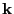-mesh) inside the Brillouin zone. One way to rectify this problem is to approximate the step-function occupation function wit a Fermi-Dirac function of finite temperature. This makes the integrand a differentiable function everywhere in the Brillouin zone and thus improves-mesh convergence though one has to live with the fact that the result obtained is actually for a slightly different problem of system under finite temperatures. In fact one can show that by simply approximating the step function with a Fermi-Dirac distribution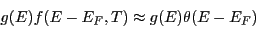where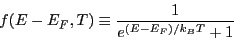we in general cannot get the integral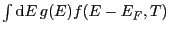to equal toexactly, this can be seen clearly in figure 3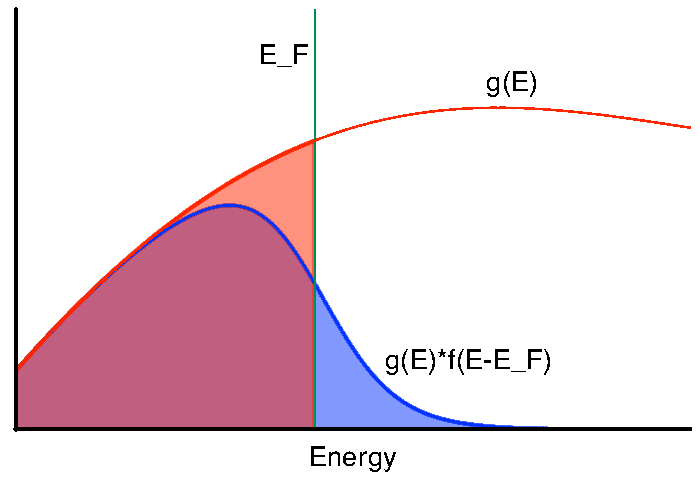A more sophisticated approximation to the occupation function can be done using Methfessel and Paxton method. This method approximates the step-function by starting with approximating the delta-function using expansion in a set of orthogonal Hermite polynomials. And then the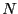-th order approximation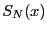is obtained by integrating the delta function. The method guarantees that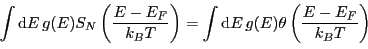for any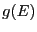that is an polynomial of-th order or less. The expansion in Hermite polynomials are given as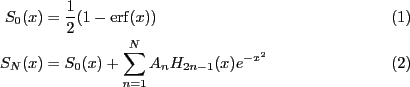where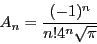(4)

and Hermite polynomials can be generated by the recurrence relationNote that at 0-th order, the Methfessel-Paxton method corresponds to a simple Fermi-Dirac like smearing.

The ground-state energy when calculated using Methfessel-Paxton smearing is no-longer variational. Instead the ground-state density minimises the free energy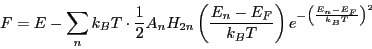(5)

Difference between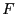and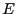are small even for large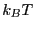. This makes Methfessel-Paxton method desirable for calculations with metallic systems, one will be able to use a much corser reciprocal grid with a relatively high smearing factorwithout introducing too much difference betweenand. If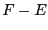is large then it means the calculated ground-state energy deviates from the true zero-temperature energy--which we are trying to approximate--greatly and therefore the result is unreliable.

Figure 4: Left: Methfessel-Paxton approximation to delta function. Right: Methfessel approximation to step function.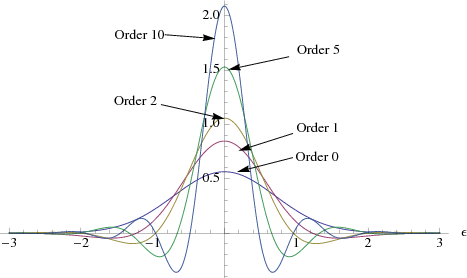However Methfessel-Paxton method also introduces a numerical artefact that Fermi-energy may no-longer be unique. To see this we notice that by expanding the delta function in terms Hermite polynomials, we introduce a number of roots to the function, and this translates into regions of negative occupancies in. This is illustrated in figure 4. As a result, the electron number as a function of Fermi energy: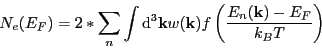(6)

(whereis weight, and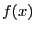is the occupation function, which is given asfor orderMethfessel-Paxton approximation) is no-longer monotonic. This behaviour can be illustrated by a simple toy model where we imaging have a system with the density of states represented as two gaussian function separated by some energy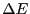, then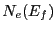can be calculated easily and are plotted in figure 5. As we can see, there is a possibility that for a given number of electrons there are more than one Fermi energies that would give the correct answer.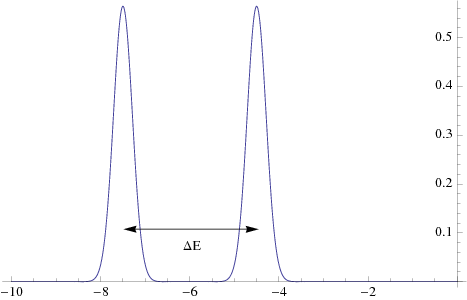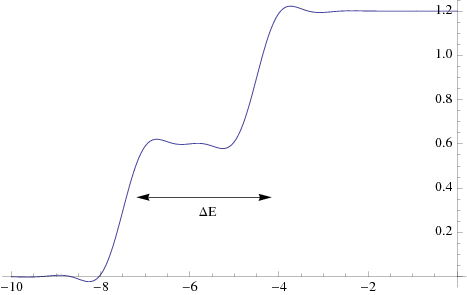While given a lower bound and upper bound in functionthe bisection method always returns a solutionthat gives the desired electron numbers, it is important that we are consistent on which--if there are more than one--we actually find. Note that the properties of non-unique Fermi energy is not physical, but is an artefact of Methfessel-Paxton approximation. Never the less, to be consistent in our calculations we define the Fermi energy to be always the lowest energy that give the correct electron number.

To make sure we always find the lowest energy solution, we need to find the lower and upper energy bounds very carefully. To get a lower bound, we could always start from the lowest energy. However to be more efficient, this energy is probably too low, and we define a parameter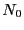such that we fill up the lower bands (and assuming occupation of 1.0) until we have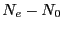electrons. And we use the highest filled energy as the lower bound. To find the upper bound, we increase the lower band by energy steps of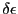untilis greater than the desired number of electrons. To find, we have to be careful the that it is not too big and miss one possible solution and at the same time not too small so that calculation is still efficient. We note that the width of the Methfessel-Paxton approximation to delta function is controlled by the gaussian weight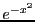, where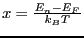. Hence we may define the width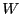of the Methfessel-Paxton approximation to delta function as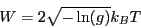(7)

where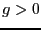is a user definable parameter. Then we prove that--see theorem A.1--for a Methfessel-Paxton approximation of order, there are exactlyroots for--Methfessel-Paxton approximation to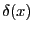. This implies we can choose the energy step to be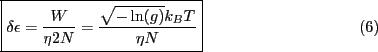where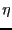is a user definable parameter controlling finess of the step. If,should be small enough to only go over one stationary point inat a time--rememberis the derivative of. And hence if starting from an absolute lower bound, the next upper bound we will find forwill guaranteed to bracket only the lowest Fermi Energy. We can then use the bisection method to find.

Subsections
Lianheng Tong 2011-03-02